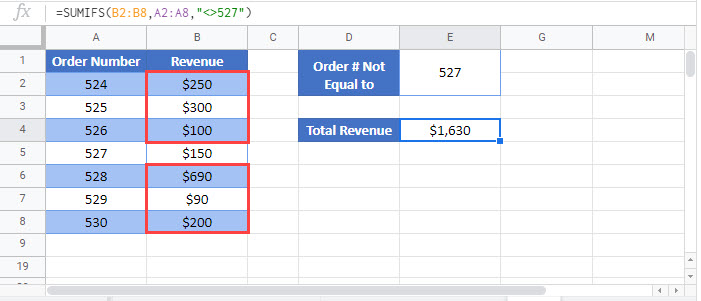# Sum If Cells Not Equal to – Excel & Google Sheets

This tutorial will demonstrate how to use the SUMIFS Function to sum rows not equal to specific values in Excel and Google Sheets.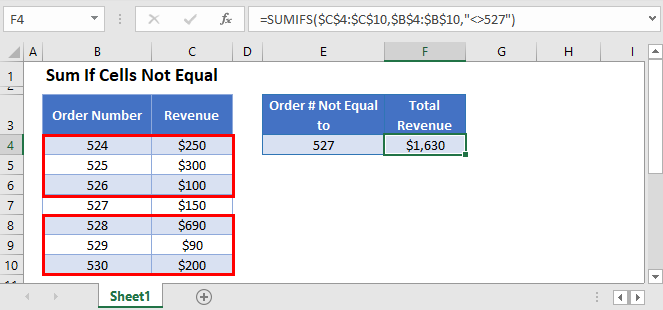## Sum If Not Equal To

The SUMIFS Function sums data that meet certain criteria. Its syntax is: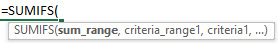This example will sum the Revenue for all Order Numbers not equal to 527.

``=SUMIFS(C3:C9,B3:B9,"<>527")``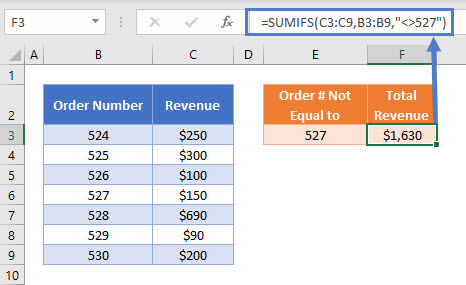As shown above, to test whether the Order Number is not equal to 527, we use:

``"<>527"``

Note that when hard-coding the criteria into the SUMIFS Function, the logical test must be within double quotes (” “).

Other logical operators can also be used in this formula, such as:

• Equal to (“=524”)
• Greater than (“>526”)
• Greater than or equal to (“>=529”)
• Less than (“<528”)
• Less than or equal to (“<=525”)

## Sum If Not Equal To – Cell References

Usually, it is bad practice to hard-code values into formulas. Instead, it is more flexible to use a separate cell to define the criteria’s value.

``=SUMIFS(C3:C9,B3:B9,"<>"&E3)``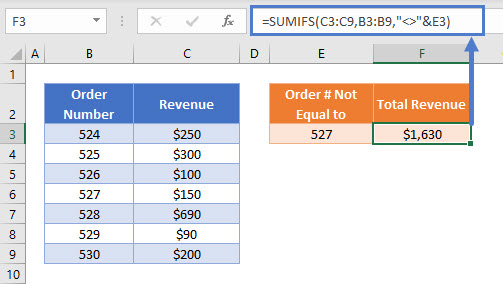Start with the logical operator within double quotes (“<>”) and use the & symbol to join the operator with the cell reference:

``"<>"&E3``

## Locking Cell References

To make our formulas easier to read, we’ve shown the formulas without locked cell references:

``=SUMIFS(C3:C9,B3:B9,"<>"&E3)``

But these formulas will not work properly when copy and pasted elsewhere in your file. Instead, you should use locked cell references like this:

``=SUMIFS(\$C\$3:\$C\$9,\$B\$3:\$B\$9,"<>"&E3)``